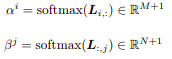# Are the following methods of calculating softmax for Attention equivalent?

I’m building modules for different reading comprehension models, BiDAF, DCN, etc.

I wanted to confirm if the following two sets of code are equivalent. The goal is to apply softmax on a similarity matrix, L, of shape (B, M+1, N+1) and calculate alpha, beta.

Where alpha and beta are defined by the following equations:Approach 1:

``````alpha = F.softmax(L, dim=2)
beta = F.softmax(L, dim=1)
beta = beta.transpose(1, 2)
``````

Approach 2:

``````alpha, beta = [ ], [ ]
for i in range(L.size(0)):
alpha.append(F.softmax(L[i],1).unsqueeze(0))
beta.append(F.softmax(L[i].transpose(0,1),1).unsqueeze(0))

alpha = torch.cat(alpha, dim=-1)
beta = torch.cat(beta, dim=-1)
``````

I think the first approach is more efficient, as it doesn’t use a for loop.

Would really appreciate any advice on this!